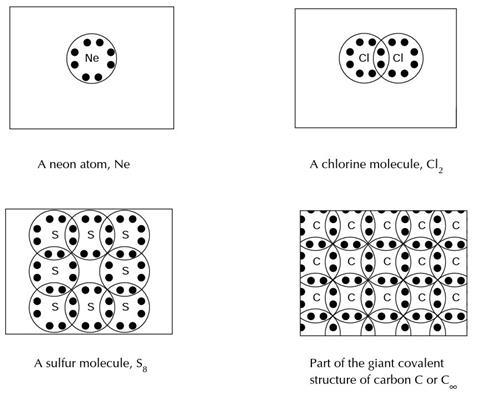It is known that students may have difficulty in distinguishing between substances with simple molecules, and those with giant covalent lattices

This exercise is intended to make students think about this issue, and to realize that although diamond is commonly given the formula ‘C’, this should not be taken to imply it forms monatomic molecules.

## Predicting the melting temperature of carbon

Part of doing science involves spotting patterns, and making predictions which can be tested by experiments. In this exercise you will be asked to make a prediction based on information you will be given. It is not important whether your prediction is correct, as long as it is based on the information given.

Many substances are said to be ‘molecular’ - they are comprised of vast numbers of separate identical particles. The name given to the tiny particles in these substances is molecules. Usually a molecule can be thought of as several atoms bonded together. In a few substances the molecules are single atoms. Often these substances are called atomic substances.

The formula of helium is He. It consists of single atoms. The relative atomic mass of helium, Ar (He), is 4.0, and its relative molecular mass, Mr (He), is also 4.0.

The formula of fluorine is F2. It has molecules that can be thought of as two atoms bonded together. The relative atomic mass of fluorine, Ar (F), is 19.0, and its relative molecular mass, Mr (F2), is therefore 38.0.

The formula of sulfur is S8. It has molecules that can be thought of as eight atoms bonded in a ring arrangement. The relative atomic mass of sulfur, Ar(S), is 32.1, and its relative molecular mass, Mr(S8), is therefore 256.8.

1. The formula of neon is Ne. It consists of single atoms. The relative atomic mass of neon, Ar (Ne) is 20.2. What is the relative molecular mass of neon? Mr (Ne) = ?
2. The formula of chlorine is Cl2. It has molecules that that can be thought of as two atoms bonded together. The relative atomic mass of chlorine, Ar(Cl), is 35.5. What is the relative molecular mass of chlorine? Mr(Cl2) = ?
3. The formula of carbon is C. The relative atomic mass of C is 12.0. What do you think the relative molecular mass of carbon will be? Mr (C) = ?

The table below shows the melting temperatures (in Kelvin, K) of some elements.

Element Mr Melting temperature /K
Helium (He)  4.0  4
Carbon (C)  12.0
Neon (Ne)  20.2  25
Fluorine (F2)  38.0  53
Chlorine (Cl2)  71.0  172
Bromine (Br2)  159.8  266
Iodine (I2)  253.8  387
Sulphur (S8)  256.8  392

It has been suggested that there is a general relationship between the relative molecular mass of an element, and the temperature at which the solid element melts.

1. Can you see any relationship in the data given in the table? Describe any pattern you can see:
2. Predict the (approximate) melting temperature for carbon? Make an estimate, and explain your reasons: Solid carbon will melt at about …? I think this because …?

## Explaining the melting temperature of carbon

Write your prediction of the approximate melting temperature of carbon.

This is your prediction based upon the data you were given. In science it is important to make predictions, because while testing the predictions we are also testing the ideas we use to explain things. Often when our predictions are wrong it helps us find better ways of understanding things.

Experiments show that solid carbon is difficult to melt and only changes into a liquid at a very high temperature. The melting temperature of carbon is 3823K. How close was your prediction?

Below you will find four diagrams showing the particles in neon, chlorine, sulfur and carbon. It is not possible to draw accurate diagrams to show exactly what atoms and molecules are like. These picture are very simple models of how scientists sometimes think about these particles.2. Scientists sometimes describe carbon as having a giant covalent structure. What do you think is meant by giant covalent?
3. It is sometimes suggested that the symbol for the carbon macromolecule should be C rather than just C. What do you think the ∞ symbol is meant to show in C?

### Predicting the melting temperature of carbon

1. Mr (Ne) = 1 x 20.2 = 20.2
2. Mr (Cl2) = 2 x 35.5 = 71.0
3. Mr (C) = 1 x 12.0 = 12.0
1. Students should recognise that in the examples given, melting temperature increases with relative molecular mass.
2. The students should predict a melting temperature greater than 4K and less than 25K – and explain that they have selected an estimate to have a higher melting temperature than helium, and a lower melting temperature than neon.

### Explaining the melting temperature of carbon

1. The diagrams should help explain the melting temperature of carbon because: unlike the other examples (neon, chlorine and sulfur) carbon does not comprise of separate (ie discrete) molecules, but a large (ie extensive) structure (lattice). Melting carbon requires breaking covalent bonds - not just overcoming intermolecular forces.
2. Giant covalent is meant to suggest: that the structure of carbon comprises of a very large number of units all interconnected through strong covalent bonds.
3. The symbol ∞ is meant to suggest either that the structure may be considered as effectively infinite or that a single carbon atom should not be considered to be a molecule of carbon.